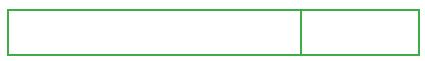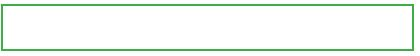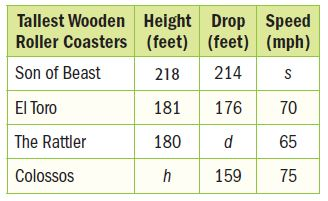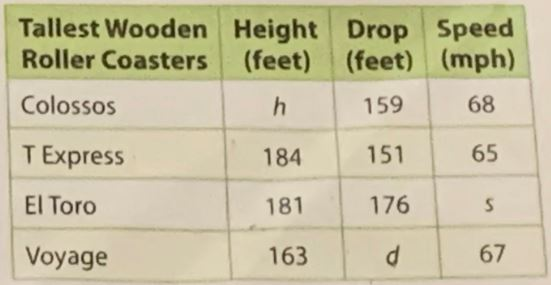Homework Explained - Math Practice 101Dear guest, you are not a registered member. As a guest, you only have read-only access to our books, tests and other practice materials.

As a registered member you can:

Registration is free and doesn't require any type of payment information. Click here to Register.
Go to page:
Chapter 6: Equations and Inequalities; Lesson 1: One-Step Addition And Subtraction Equations

Solve each equation. Check your solution.

• Question 1

$$a + 3 = 10$$

• Question 2

$$y + 5 = -11$$

• Question 3

$$s - 8 = 9$$

• Question 4

$$5 = x + 8$$

• Question 5

$$-2 = p - 1$$

• Question 6

$$14 = s + 7$$

Use a bar diagram to solve arithmetically. Then use an equation to solve algebraically.

• Question 7

Last week Tiffany practiced her bassoon a total of 7 hours. This was 2 hours more than she practiced the previous week. How many hours did Tiffany practice the previous week?• Question 8• Question 9

Multiple Representations Use the table to solve.
a. Symbols The difference in speeds of Son of Beast and The Rattler is 13 miles per hour. If Son of Beast has the greater speed, write and solve a subtraction equation to find its speed.•  s=
• Question 9

b. Diagram The Rattler has a drop that is 52 feet less than El Toro. Draw a bar diagram to the right and write an equation to find the height of The Rattler.

•  d=
• Question 9

c. Words Let h represent the height of the Colossos roller coaster. Explain why h + 10 = 180 and h + 48 = 218 are equivalent equations. Then explain the meaning of the solution.

•  h=
• Question 10

Multiple Representations Use the table to solve.
a. Symbols The difference in speeds of El Toro and T Express is 5 miles per hour. If El Toro  has the greater speed, write and solve a subtraction equation to find its speed.•  s=
• Question 10

b. Diagram Voyage has a drop that is 22 feet less than El Toro. Draw a bar diagram to the right and write an equation to find the height of Voyage.

•  d=
• Question 10

c. Words Let h represent the height of the Colossos roller coaster. Explain why h - 13 = 184 and h - 34 = 163 are equivalent equations. Then explain the meaning of the solution.

•  h=

Yes, email page to my online tutor. (if you didn't add a tutor yet, you can add one here)